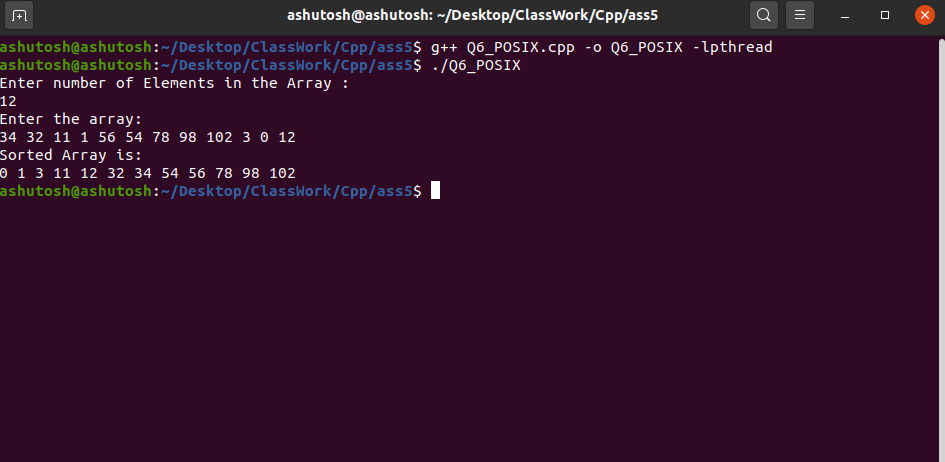Implementation of Quick sort using MPI, OMP and Posix thread

• Last Updated : 01 Nov, 2021

QuickSort is a Divide and Conquer Algorithm. It picks an element as a pivot and partitions the array around the picked pivot. There are many ways of choosing the pivot elements. They are:

• Always pick the first element as a pivot.
• Always pick the last element as the pivot (implemented below)
• Pick a random element as a pivot.
• Pick median as a pivot.

MPI: MPI stands for Message Passing Interface. Here the message is data. MPI allows data to be passed between processes in a distributed memory environment. In C, “mpi.h” is a header file that includes all data structures, routines, and constants of MPI. Using “mpi.h” parallelized the quick sort algorithm.  Below is the C program to implement quicksort using MPI:

C

Output: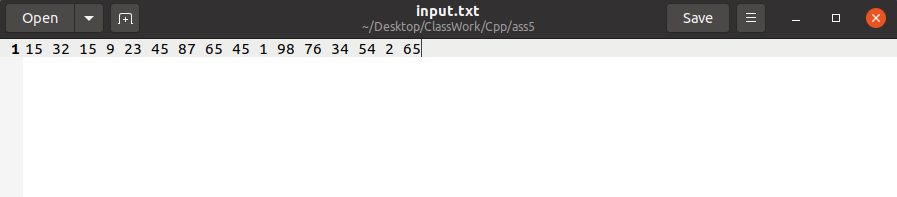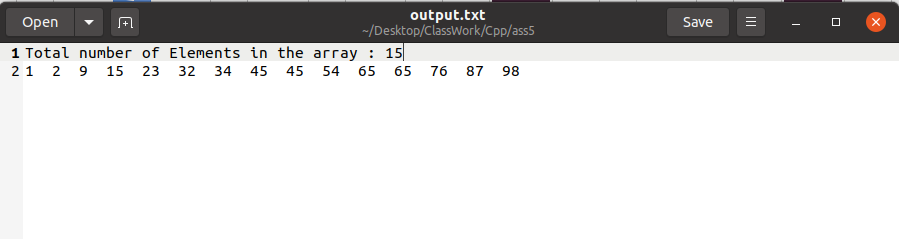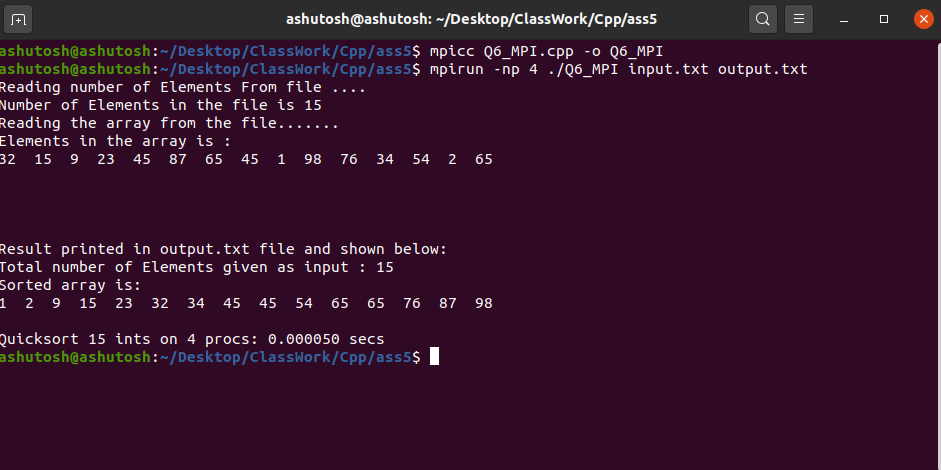OMP: OMP is Open Multi-Processing. It’s an Application Program Interface (API) that may be used to explicitly direct multi-threaded, shared memory parallelism. In C/ C++, “omp.h” is a header file that includes all the related directives related to OMP. Using “omp.h” parallelized quick sort. Below is the C++ program to implement the above concept:

C++

 // C++ program to implement the Quick Sort// using OMI#include #include using namespace std; // Function to swap two numbers a and bvoid swap(int* a, int* b){    int t = *a;    *a = *b;    *b = t;} // Function to perform the partitioning// of array arr[]int partition(int arr[], int start, int end){    // Declaration    int pivot = arr[end];    int i = (start - 1);     // Rearranging the array    for (int j = start; j <= end - 1; j++) {        if (arr[j] < pivot) {            i++;            swap(&arr[i], &arr[j]);        }    }    swap(&arr[i + 1], &arr[end]);     // Returning the respective index    return (i + 1);} // Function to perform QuickSort Algorithm// using openmpvoid quicksort(int arr[], int start, int end){    // Declaration    int index;     if (start < end) {         // Getting the index of pivot        // by partitioning        index = partition(arr, start, end); // Parallel sections#pragma omp parallel sections        {#pragma omp section            {                // Evaluating the left half                quicksort(arr, start, index - 1);            }#pragma omp section            {                // Evaluating the right half                quicksort(arr, index + 1, end);            }        }    }} // Driver Codeint main(){    // Declaration    int N;     // Taking input the number of    // elements we wants    cout << "Enter the number of elements"         << " you want to Enter\n";    cin >> N;     // Declaration of array    int arr[N];     cout << "Enter the array: \n";     // Taking input that array    for (int i = 0; i < N; i++) {        cin >> arr[i];    }     // Calling quicksort having parallel    // code implementation    quicksort(arr, 0, N - 1);     // Printing the sorted array    cout << "Array after Sorting is: \n";     for (int i = 0; i < N; i++) {        cout << arr[i] << " ";    }     return 0;}

Output: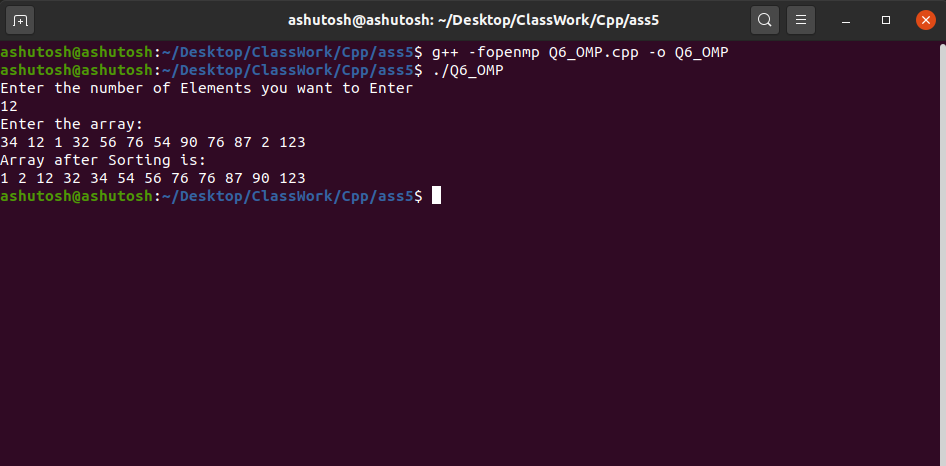POSIX Threads: The POSIX thread libraries are a C/C++ thread API based on standards. It enables the creation of a new concurrent process flow. It works well on multi-processor or multi-core systems, where the process flow may be scheduled to execute on another processor, increasing speed through parallel or distributed processing. Below is the C++ program to implement quicksort using POSIX threads:

C++14